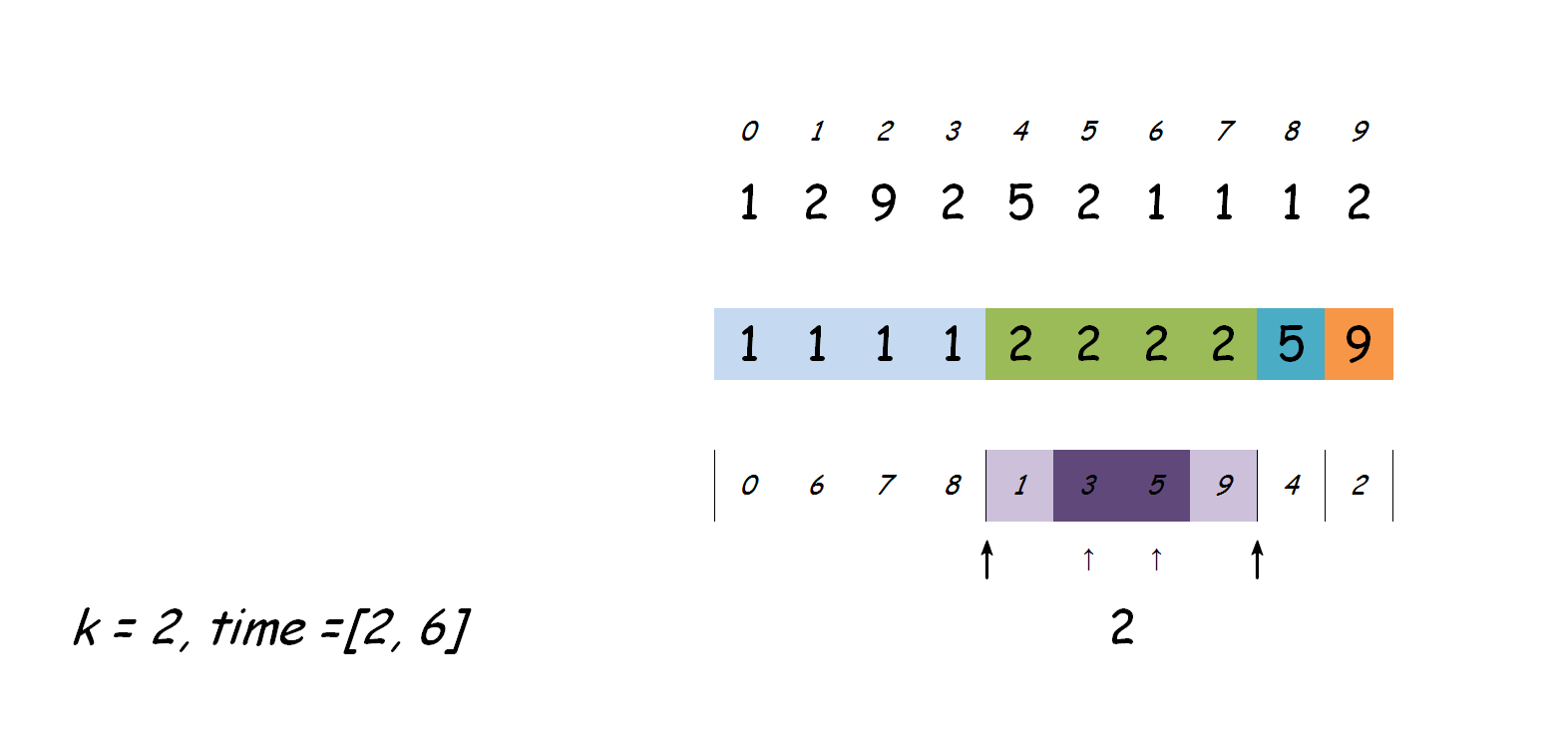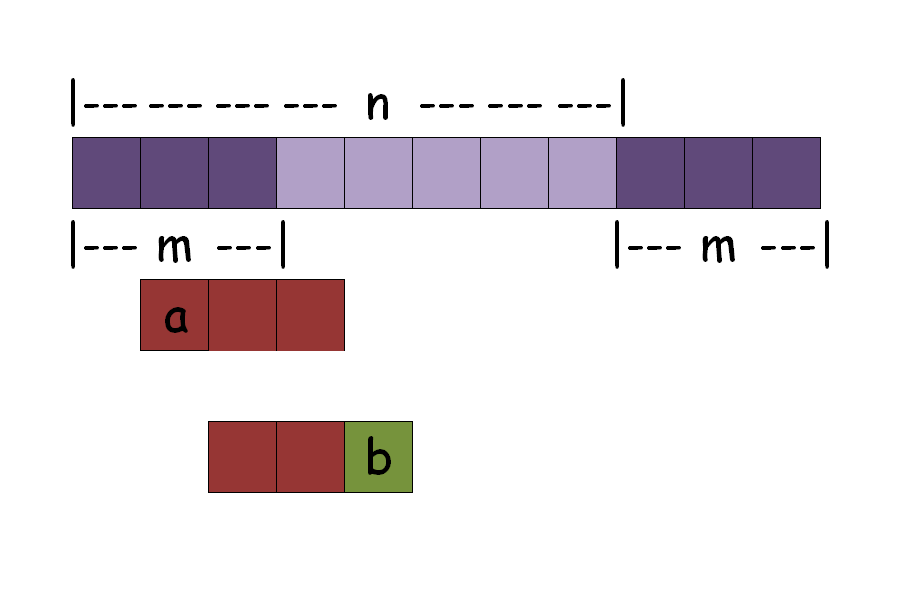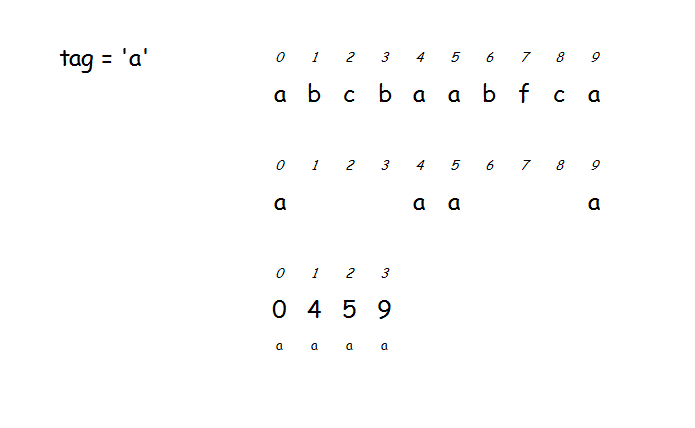# 第一题：用户喜好

### 输出：

#### 样例输入：

5
1 2 3 3 5
3
1 2 1
2 4 5
3 5 3


#### 样例输出：

1
0
2


## 解析

bool sort_cmp(const pair<int, int> &A, const pair<int, int> &B)
{
return A.first == B.first ? A.second < B.second :
A.first < B.first;
}equal_rangelower_boundupper_bound这三个函数的时间复杂度都为 O(logn) O ( l o g n ) <script type="math/tex" id="MathJax-Element-210">O(logn)</script>，因此问题得到解决。

## 代码

#include <bits/stdc++.h>

using namespace std;

bool sort_cmp(const pair<int, int> &A, const pair<int, int> &B)
{
return A.first == B.first ? A.second < B.second :
A.first < B.first;
}

struct find_first_cmp {
bool operator()(const pair<int, int> &P, int k) const
{
return P.first < k;
}

bool operator()(int k, const pair<int, int> &P) const
{
return k < P.first;
}
};

struct find_second_cmp {
bool operator()(const pair<int, int> &P, int k) const
{
return P.second < k;
}

bool operator()(int k, const pair<int, int> &P) const
{
return k < P.second;
}
};

int main()
{
int n, q;
while (EOF != scanf("%d", &n)) {
vector<pair<int, int> > arr;
for (int i = 0, x; i < n; cin >> x, arr.emplace_back(x, ++i)) {}
sort(arr.begin(), arr.end(), sort_cmp);

for (scanf("%d", &q); q--;) {
int L, R, k;
scanf("%d%d%d", &L, &R, &k);
pair<vector<pair<int, int> >::iterator, vector<pair<int, int> >::iterator> sd =
equal_range(arr.begin(), arr.end(), k, find_first_cmp{});
printf("%d\n", upper_bound(sd.first, sd.second, R, find_second_cmp{}) -
lower_bound(sd.first, sd.second, L, find_second_cmp{}));
}
}
return 0;
}


# 第二题：手串

### 输出：

#### 样例输入：

5 2 3
3 1 2 3
0
2 2 3
1 2
1 3


#### 样例输出：

2


## 解析## 代码

#include <bits/stdc++.h>

using namespace std;

int main()
{
int n, m, c;
while (EOF != scanf("%d%d%d", &n, &m, &c)) {
vector<vector<int> > arr(n + m);
for (int i = 0, num, x; i < n; i++)
for (scanf("%d", &num); num--;
arr[i].push_back((scanf("%d", &x), x))) {}
for (int i = 0; i < m; i++)
arr[n + i] = arr[i];
vector<bool> used(c, false);
map<int, int> color;
for (int i = 0; i < c; color[i++] = 0) {}

for (int i = 0; i < n + m; i++) {
for (int j = 0; !(i < m) && j < (int)arr[i - m].size(); j++)
color[arr[i - m][j] - 1]--;
for (int j = 0; j < (int)arr[i].size(); j++)
color[arr[i][j] - 1]++;
for (map<int, int>::iterator it = color.begin(); it != color.end(); ++it)
used[it->first] = it->second > 1 ? true : used[it->first];
}
int ans = 0;
for (int i = 0; i < c; ans += used[i++]) {}
printf("%d\n", ans);
}
return 0;
}


# 第三题：字母交换

### 输出：

#### 样例输入：

abcbaa 2


#### 样例输出：

2


## 解析## 代码

#include <bits/stdc++.h>

using namespace std;

int main()
{
string s;
int m;
while (cin >> s >> m) {
int ans = 1;
for (char c = 'a'; c <= 'z'; c++) {
vector<int> pos;
for (int i = 0; i < (int)s.size(); i++)
if (c == s[i])
pos.push_back(i);

if (pos.size() < 2)
continue;

int ret = 1;
vector<vector<int> > dp(pos.size(), vector<int>(pos.size(), 0));

for (int len = 2; len <= (int)pos.size(); ++len) {
for (int i = 0; i + len - 1 < (int)pos.size(); i++) {
dp[i][i + len - 1] = dp[i + 1][i + len - 2] + pos[i + len - 1] - pos[i] - len + 1;
if (dp[i][i + len - 1] <= m)
ret = len;
}
}
ans = max(ans, ret);
}
cout << ans << endl;
}
return 0;
}

08-24134508-221437
10-171万+
02-082040
04-233800
03-151万+
08-2710万+
05-094万+
06-096万+